### Home > INT3 > Chapter Ch11 > Lesson 11.1.1 > Problem11-8

11-8.
1.In Lesson 11.1.2 you will focus on multiplying and dividing rational expressions. Recall what you learned about multiplying and dividing fractions in a previous course as you answer the questions below. To help you, the following examples have been provided. Homework Help ✎

2.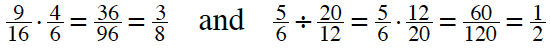1. Without a calculator, multiply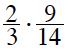and reduce the result. Describe your method for multiplying fractions.

2. Without a calculator, divide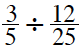and reduce the result. Then use a calculator to check your answer. Describe your method for dividing fractions.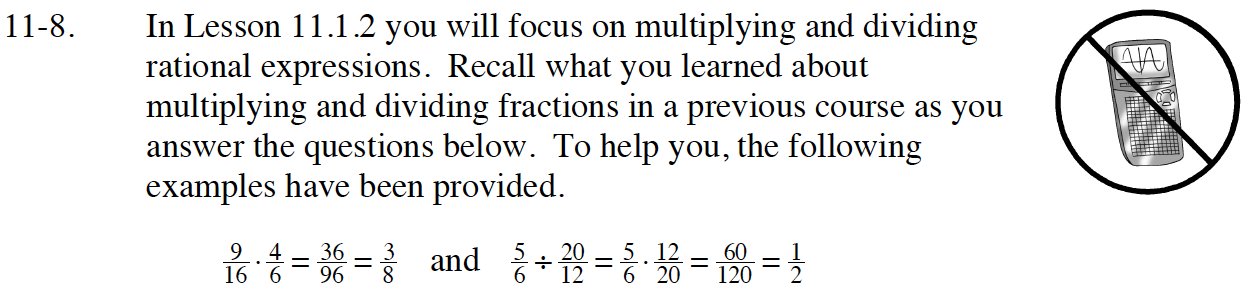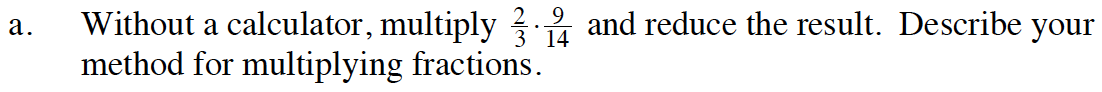Multiply the numerators together to find the numerator of the final answer, and multiply the denominators together to find the denominator of the final answer.

Do 2 and 14 have a common factor? Do 3 and 9 have a common factor? It is possible to reduce before multiplying across? Use whichever method is easiest for you.

$\frac{3}{7}$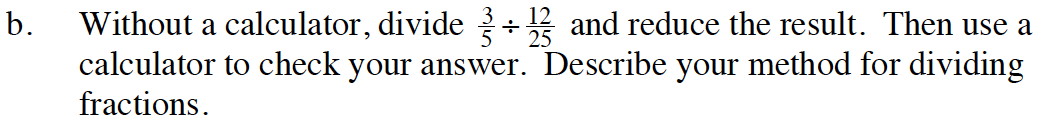Take the reciprocal of the divisor, and multiply the two resulting fractions.